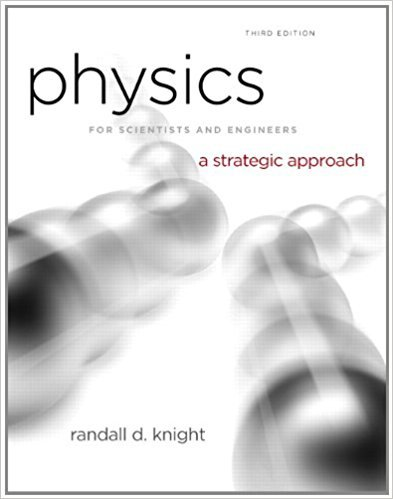×
Get Full Access to Physics For Scientists And Engineers: A Strategic Approach With Modern Physics - 3 Edition - Chapter 6 - Problem 66p
Get Full Access to Physics For Scientists And Engineers: A Strategic Approach With Modern Physics - 3 Edition - Chapter 6 - Problem 66p

×

# Very small objects, such as dust particles, experience aISBN: 9780321740908 69

## Solution for problem 66P Chapter 6

Physics for Scientists and Engineers: A Strategic Approach with Modern Physics | 3rd Edition

• Textbook Solutions
• 2901 Step-by-step solutions solved by professors and subject experts
• Get 24/7 help from StudySoup virtual teaching assistantsPhysics for Scientists and Engineers: A Strategic Approach with Modern Physics | 3rd Edition

4 5 1 301 Reviews
11
5
Problem 66P

Problem 66P

Very small objects, such as dust particles, experience a linear drag force,= (bv, direction opposite the motion), where b is a constant. That is, the quadratic model of drag of Equation 6.16 fails for very small particles. For a sphere of radius R, the drag constant can be shown to be b = 6πηR, where η is the viscosity of the gas.

a. Find an expression for the terminal speed vterm of a spherical particle of radius R and mass mfalling through a gas of viscosity η.

b. Suppose a gust of wind has carried a 50-μm-diameter dust particle to a height of 300 m. If the wind suddenly stops, how long will it take the dust particle to settle back to the ground? Dust has a density of 2700 kg/m3, the viscosity of 25°C air is 2.0 × 10−5 N s/m2, and you can assume that the falling dust particle reaches terminal speed almost instantly.

Step-by-Step Solution:

Solution 66 P

a.)

Step 1 of 5

We have to find an expression for the terminal speedof a spherical particle of radiusand massfalling through a gas of viscosity.

The speed at which the exact balance between the upward drag force and the downward

gravitational force causes an object to fall without acceleration is called the terminal speedWhere,Drag forcegravitational force

Step 2 of 5

Step 3 of 5

##### ISBN: 9780321740908

Unlock Textbook Solution